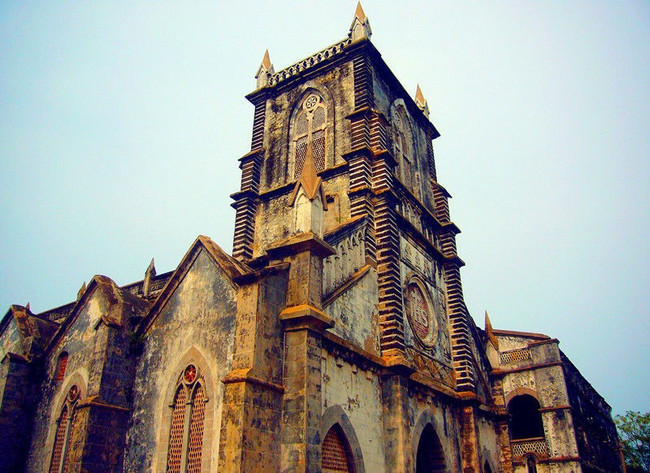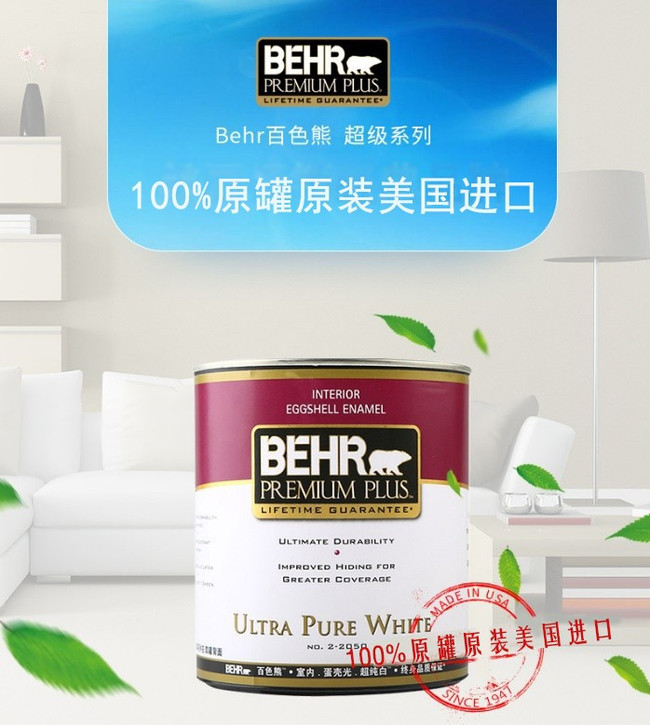|

# 美国信用体系下诞生的不仅有诚信 还有百色熊

论诚信，必须要提到美国。美国是世界上公认的信用体系建设强国，美国的个人信用体系有着悠久的发展历史、高速的运行效率以及严谨的监督体制。信用体系的公正性，确保了美国的经济繁荣以及American dream。那么今天，我们就从宗教、法律、教育、舆论、征信五个机构体系，来给各位科普一下让人肃然起敬的美国信用体系。

宗教

圣经十诫有“不可作假证陷害人”；佛曰“不妄语”；伊斯兰教倡导“骗子不是我的……

可见宗教对诚信的要求十分严格，几乎每个宗教教义对个人诚信都有明确的要求，对于虔诚的教众而言，宗教戒律对个人的诚信十分具有约束力。

在美国，宗教尤其是基督教，对美国的诚信氛围影响巨大。调查显示，接近80%的美国人属于基督教的各种教派，在美国人的行为准则里，圣经如同道德准则，甚至等同于法律。值得关注的是，美国的立国之本，就是以圣经为基础的！

法律

法律对整个社会的诚信形成起着强制性的作用。而美国单信用管理的相关法律便有16项，这些法案构成了美国国家信用体系正常运转的法律环境。

为了保证信息公开，美国还颁布了一系列的法律条文，一方面保证了与信用信息有关的信息披露的公开、透明，另一方面又在法律上理清了公开信息与国家秘密、商业秘密及个人秘密的关系，使信用调查机构可以合法地获得大量的信用信息。教育

诚信要从娃娃抓起，美国从幼儿园和小学起就重视对孩子的诚信教育，而这十几年的教育不仅形成了美国人的个人习惯，也形成了整个美国社会的诚信氛围。

所以大部分美国人长大后，对守信行为都是持一种很自然的肯定态度。而且在美国，警察或法定人员有相当大的权利，越红灯、超速的罚单是在350-1000美元以上的罚款。所以大部分美国孩子，除了从小被教育为比较诚信外也比较守法。如此一来，就能形成一个他律和自律相结合的良好信用环境。

舆论

新闻媒体是继立法、司法和行政之后的“第四”项权力，新闻记者被称为“无冕之王”。美国新闻媒体的报道是自由和无孔不入的，它就好像是一双眼睛，总是在无时无刻盯着你。

作为第三方信号发送者，新闻媒体对美国的社会诚信以及征信机构起到了监督的作用，对维持社会健康运转也起到了重要作用。人们不仅处于法律的监督之下，而且还置于新闻媒体织就的“舆论之网”中，接受现代媒体的言语、声音、图象等自身潜移默化的控制。作为社会大众感受最新事件的眼睛和口舌，美国新闻媒体的重要性不言而喻。

征信

与新闻媒体不同的是，征信机构通过收集交易个体的信息，构建个体数据库，用累计的客观事实统计记录作为个体评级的依据。可以说，征信机构比你自己还要了解你自己。

对于失信的企业或个人，征信机构将会通过信用调查来依法提供、传播其不良信用记录和低水平的信用评估，使他们失去商业上的机会或提高其交易成本，从而抑制失信行为的发生。由于信用交易与个人的日常生活密切相关，美国的消费者都十分注重自身的信用状况，并会定期向信用局查询自己的信用报告，尽可能避免在信用局的报告出现自己的负面信息。

BEHR百色熊™

在如此严格的信用体系下，必然诞生世界上最权威的室内空气质量认证，那就是美国绿色卫士GREENGUARD环保认证。GREENGUARD是全球专注于室内空气质量认证的机构，其发布的金牌认证，提供更严格的认证标准，以确保认证的产品可用于学校和医院等环保要求极高的场所。

在涂料品牌中，BEHR百色熊®是全球首家取得认证的公司之一。根据认证，BEHR百色熊®的PP(超级系列)、PPUI(纳米科技系列)系列产品均适用儿童房，基本在涂刷当天就没有味道了。

美国加州南海岸空气品质管理局（South Coast AirQuality Management District，SCAQMD）针对建筑涂料产品的VOC 含量及排放，进行严格限定。此限定标准目前是全美最为严格的VOC（50克/升）限定要求，远高于美国国家环境保护署EPA 制定的联邦政府标准。若任何涂料厂商在卖场被检查到不符合规格的产品，罚款将高达一天1百万美元。

就如之前提到的，这是一个“重罚”的国家。这也是因为这是一个保护消费者权利很高的国家。目前所有百色熊出到美国的货都是从加州Santa Ana厂房生产的，也就是务必符合美国加州南海岸空气品质管理局规定的城市。自1947年创立以来，BEHR百色熊®始终信奉环保为上的品牌价值，70年来从未随时间而改变。在即将到来的“315”这个特殊的日子里，BEHR百色熊®带着满满的诚意，邀您参加BEHR百色熊®线下体验活动，共同见证诚信的力量！马上来BEHR百色熊®线下门店，就能参与各种力度超大的优惠活动。除此之外，还有机会获赠幸运大奖！活动期间，还可参与免费试刷体验，还等什么？赶快来参加吧！

`声明：本文由入驻焦点开放平台的作者撰写，除焦点官方账号外，观点仅代表作者本人，不代表焦点立场错误信息举报电话： 400-099-0099，邮箱：jubao@vip.sohu.com，或点此进行意见反馈，或点此进行举报投诉。`A B C D E F G H J K L M N P Q R S T W X Y Z
A - B - C - D - E
• A
• 鞍山
• 安庆
• 安阳
• 安顺
• 安康
• 澳门
• B
• 北京
• 保定
• 包头
• 巴彦淖尔
• 本溪
• 蚌埠
• 亳州
• 滨州
• 北海
• 百色
• 巴中
• 毕节
• 保山
• 宝鸡
• 白银
• 巴州
• C
• 承德
• 沧州
• 长治
• 赤峰
• 朝阳
• 长春
• 常州
• 滁州
• 池州
• 长沙
• 常德
• 郴州
• 潮州
• 崇左
• 重庆
• 成都
• 楚雄
• 昌都
• 慈溪
• 常熟
• D
• 大同
• 大连
• 丹东
• 大庆
• 东营
• 德州
• 东莞
• 德阳
• 达州
• 大理
• 德宏
• 定西
• 儋州
• 东平
• E
• 鄂尔多斯
• 鄂州
• 恩施
F - G - H - I - J
• F
• 抚顺
• 阜新
• 阜阳
• 福州
• 抚州
• 佛山
• 防城港
• G
• 赣州
• 广州
• 桂林
• 贵港
• 广元
• 广安
• 贵阳
• 固原
• H
• 邯郸
• 衡水
• 呼和浩特
• 呼伦贝尔
• 葫芦岛
• 哈尔滨
• 黑河
• 淮安
• 杭州
• 湖州
• 合肥
• 淮南
• 淮北
• 黄山
• 菏泽
• 鹤壁
• 黄石
• 黄冈
• 衡阳
• 怀化
• 惠州
• 河源
• 贺州
• 河池
• 海口
• 红河
• 汉中
• 海东
• 怀来
• I
• J
• 晋中
• 锦州
• 吉林
• 鸡西
• 佳木斯
• 嘉兴
• 金华
• 景德镇
• 九江
• 吉安
• 济南
• 济宁
• 焦作
• 荆门
• 荆州
• 江门
• 揭阳
• 金昌
• 酒泉
• 嘉峪关
K - L - M - N - P
• K
• 开封
• 昆明
• 昆山
• L
• 廊坊
• 临汾
• 辽阳
• 连云港
• 丽水
• 六安
• 龙岩
• 莱芜
• 临沂
• 聊城
• 洛阳
• 漯河
• 娄底
• 柳州
• 来宾
• 泸州
• 乐山
• 六盘水
• 丽江
• 临沧
• 拉萨
• 林芝
• 兰州
• 陇南
• M
• 牡丹江
• 马鞍山
• 茂名
• 梅州
• 绵阳
• 眉山
• N
• 南京
• 南通
• 宁波
• 南平
• 宁德
• 南昌
• 南阳
• 南宁
• 内江
• 南充
• P
• 盘锦
• 莆田
• 平顶山
• 濮阳
• 攀枝花
• 普洱
• 平凉
Q - R - S - T - W
• Q
• 秦皇岛
• 齐齐哈尔
• 衢州
• 泉州
• 青岛
• 清远
• 钦州
• 黔南
• 曲靖
• 庆阳
• R
• 日照
• 日喀则
• S
• 石家庄
• 沈阳
• 双鸭山
• 绥化
• 上海
• 苏州
• 宿迁
• 绍兴
• 宿州
• 三明
• 上饶
• 三门峡
• 商丘
• 十堰
• 随州
• 邵阳
• 韶关
• 深圳
• 汕头
• 汕尾
• 三亚
• 三沙
• 遂宁
• 山南
• 商洛
• 石嘴山
• T
• 天津
• 唐山
• 太原
• 通辽
• 铁岭
• 泰州
• 台州
• 铜陵
• 泰安
• 铜仁
• 铜川
• 天水
• 天门
• W
• 乌海
• 乌兰察布
• 无锡
• 温州
• 芜湖
• 潍坊
• 威海
• 武汉
• 梧州
• 渭南
• 武威
• 吴忠
• 乌鲁木齐
X - Y - Z
• X
• 邢台
• 徐州
• 宣城
• 厦门
• 新乡
• 许昌
• 信阳
• 襄阳
• 孝感
• 咸宁
• 湘潭
• 湘西
• 西双版纳
• 西安
• 咸阳
• 西宁
• 仙桃
• 西昌
• Y
• 运城
• 营口
• 盐城
• 扬州
• 鹰潭
• 宜春
• 烟台
• 宜昌
• 岳阳
• 益阳
• 永州
• 阳江
• 云浮
• 玉林
• 宜宾
• 雅安
• 玉溪
• 延安
• 榆林
• 银川
• Z
• 张家口
• 镇江
• 舟山
• 漳州
• 淄博
• 枣庄
• 郑州
• 周口
• 驻马店
• 株洲
• 张家界
• 珠海
• 湛江
• 肇庆
• 中山
• 自贡
• 资阳
• 遵义
• 昭通
• 张掖
• 中卫

1室1厅1厨1卫1阳台

1
2
3
4
5

0
1
2

1

1

0
1
2
3报名成功，资料已提交审核A B C D E F G H J K L M N P Q R S T W X Y Z
A - B - C - D - E
• A
• 鞍山
• 安庆
• 安阳
• 安顺
• 安康
• 澳门
• B
• 北京
• 保定
• 包头
• 巴彦淖尔
• 本溪
• 蚌埠
• 亳州
• 滨州
• 北海
• 百色
• 巴中
• 毕节
• 保山
• 宝鸡
• 白银
• 巴州
• C
• 承德
• 沧州
• 长治
• 赤峰
• 朝阳
• 长春
• 常州
• 滁州
• 池州
• 长沙
• 常德
• 郴州
• 潮州
• 崇左
• 重庆
• 成都
• 楚雄
• 昌都
• 慈溪
• 常熟
• D
• 大同
• 大连
• 丹东
• 大庆
• 东营
• 德州
• 东莞
• 德阳
• 达州
• 大理
• 德宏
• 定西
• 儋州
• 东平
• E
• 鄂尔多斯
• 鄂州
• 恩施
F - G - H - I - J
• F
• 抚顺
• 阜新
• 阜阳
• 福州
• 抚州
• 佛山
• 防城港
• G
• 赣州
• 广州
• 桂林
• 贵港
• 广元
• 广安
• 贵阳
• 固原
• H
• 邯郸
• 衡水
• 呼和浩特
• 呼伦贝尔
• 葫芦岛
• 哈尔滨
• 黑河
• 淮安
• 杭州
• 湖州
• 合肥
• 淮南
• 淮北
• 黄山
• 菏泽
• 鹤壁
• 黄石
• 黄冈
• 衡阳
• 怀化
• 惠州
• 河源
• 贺州
• 河池
• 海口
• 红河
• 汉中
• 海东
• 怀来
• I
• J
• 晋中
• 锦州
• 吉林
• 鸡西
• 佳木斯
• 嘉兴
• 金华
• 景德镇
• 九江
• 吉安
• 济南
• 济宁
• 焦作
• 荆门
• 荆州
• 江门
• 揭阳
• 金昌
• 酒泉
• 嘉峪关
K - L - M - N - P
• K
• 开封
• 昆明
• 昆山
• L
• 廊坊
• 临汾
• 辽阳
• 连云港
• 丽水
• 六安
• 龙岩
• 莱芜
• 临沂
• 聊城
• 洛阳
• 漯河
• 娄底
• 柳州
• 来宾
• 泸州
• 乐山
• 六盘水
• 丽江
• 临沧
• 拉萨
• 林芝
• 兰州
• 陇南
• M
• 牡丹江
• 马鞍山
• 茂名
• 梅州
• 绵阳
• 眉山
• N
• 南京
• 南通
• 宁波
• 南平
• 宁德
• 南昌
• 南阳
• 南宁
• 内江
• 南充
• P
• 盘锦
• 莆田
• 平顶山
• 濮阳
• 攀枝花
• 普洱
• 平凉
Q - R - S - T - W
• Q
• 秦皇岛
• 齐齐哈尔
• 衢州
• 泉州
• 青岛
• 清远
• 钦州
• 黔南
• 曲靖
• 庆阳
• R
• 日照
• 日喀则
• S
• 石家庄
• 沈阳
• 双鸭山
• 绥化
• 上海
• 苏州
• 宿迁
• 绍兴
• 宿州
• 三明
• 上饶
• 三门峡
• 商丘
• 十堰
• 随州
• 邵阳
• 韶关
• 深圳
• 汕头
• 汕尾
• 三亚
• 三沙
• 遂宁
• 山南
• 商洛
• 石嘴山
• T
• 天津
• 唐山
• 太原
• 通辽
• 铁岭
• 泰州
• 台州
• 铜陵
• 泰安
• 铜仁
• 铜川
• 天水
• 天门
• W
• 乌海
• 乌兰察布
• 无锡
• 温州
• 芜湖
• 潍坊
• 威海
• 武汉
• 梧州
• 渭南
• 武威
• 吴忠
• 乌鲁木齐
X - Y - Z
• X
• 邢台
• 徐州
• 宣城
• 厦门
• 新乡
• 许昌
• 信阳
• 襄阳
• 孝感
• 咸宁
• 湘潭
• 湘西
• 西双版纳
• 西安
• 咸阳
• 西宁
• 仙桃
• 西昌
• Y
• 运城
• 营口
• 盐城
• 扬州
• 鹰潭
• 宜春
• 烟台
• 宜昌
• 岳阳
• 益阳
• 永州
• 阳江
• 云浮
• 玉林
• 宜宾
• 雅安
• 玉溪
• 延安
• 榆林
• 银川
• Z
• 张家口
• 镇江
• 舟山
• 漳州
• 淄博
• 枣庄
• 郑州
• 周口
• 驻马店
• 株洲
• 张家界
• 珠海
• 湛江
• 肇庆
• 中山
• 自贡
• 资阳
• 遵义
• 昭通
• 张掖
• 中卫• 手机• 分享
• 设计
免费设计
• 计算器
装修计算器
• 入驻
合作入驻
• 联系
联系我们
• 置顶
返回顶部